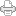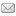Posted on Tuesday, November 22, 2011 at 06:23AM

As we make the course correction discussed in the entry below, it’s useful to note that we can now view Einstein’s mass -> energy equation in terms of the SUDR/TUDR combos.

To see this, we need only change the way the equation is usually written. We change from

E = mc2,

to

E = c2 * m,

which, in space/time terms, may be written,

t/s = (s/t)2 * (t/s)3,

but this can also be written in terms of SUDR and TUDR ratios, as a ratio of their respective 2D area scalar speeds to their respective 0D radius scalar speeds, in this manner:

t/s = ((s/t)/(t/s))/((s2/t)/(t2/s))

In the reciprocal speeds of the first term, the 0D radii space units yield unit speed ratios:

s/t = 2(20)/2(20) = 2/2 = 1 and t/s = 2(20)/2(20) = 2/2 = 1,

and the physical dimensions are squared, when the inverse multiplication operation is carried out:

((s/t)/(t/s)) = ((s/t)*(s/t)) = s2/t2.

However, in the 3D term, the physical dimensions are raised up from 2D to 3D, by the inverse multiplication operation:

s3/t = 2(22)/2(20) = 8/2 and t3/s = 2(22)/2(20) = 8/2.

Hence:

((s2/t)/(t2/s)) = ((s2/t)*(s/t2)) = s3/t3,

which are the volume dimensions of inverse mass.

Consequently, when the final inverse multiplication operation is carried out, we get Einstein’s familiar equation:

t/s = (s/t)2 * (t/s)3.

The surprising thing about this, however, is that the inverse  multiplication operation on the ratio of these two geometric magnitudes, equates them numerically with the next higher dimensional unit, what we might call the fundamental magnitudes of Larson’s Cube (LC), the 1D line width (21), the 2D area face (22) and the 3D volume cube (23); that is, the necessary mathematical operation of the fundamental reciprocal relation raises 2 to 4 (22 = 4) and 4 to 8 (22 * 2 = 23 = 8), and then, subsequently, the 2D area magnitude multiplied times the 3D volume magnitude is equal to the 1D line magnitude, just as it should be.

This will no doubt be really useful in working with the S|T units of the preons in terms of energy, starting with the neutrinos and working our way down to the electrons and positrons and finally combining the quarks into protons and neutrons and adding the electrons to the protons, to begin working our way up the periodic table of the elements.

But more than this, it appears that this marvelously clear insight into the meaning of the terms in Einstein’s famous equation might reduce the horribly arcane subject of modern physics to a toy model that can be taught, starting in elementary school.

Now, we need to take a look at the less famous, but more enigmatic equation of Max Planck, E = hv, in the light of the LC..View Printer Friendly VersionEmail Article to Friend